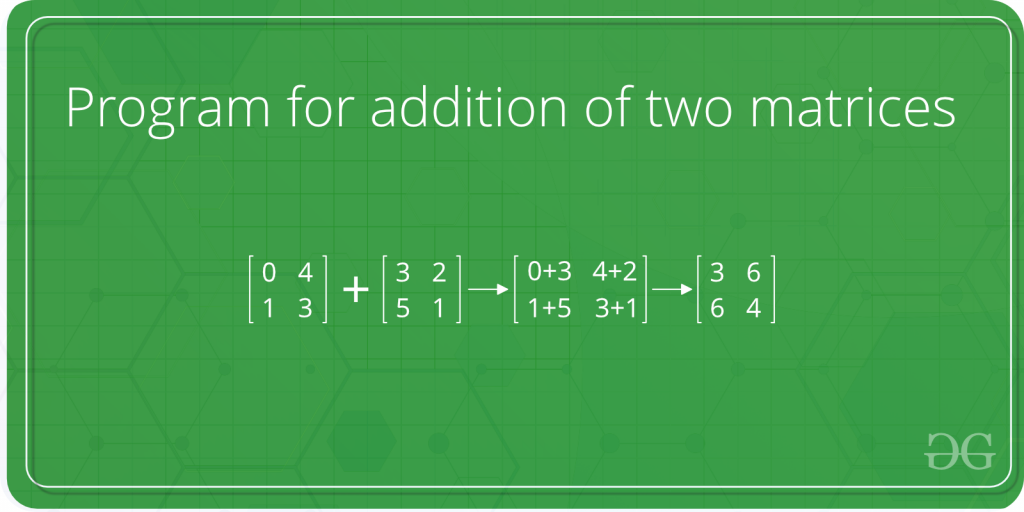Open in App
Not now

# C++ Program For Addition of Two Matrices

• Last Updated : 17 Jan, 2023

Given two N x M matrices. Find a N x matrix as the sum of given matrices each value at the sum of values of corresponding elements of the given two matrices.Approach: Below is the idea to solve the problem.

Iterate over every cell of matrix (i, j), add the corresponding values of the two matrices and store in a single matrix i.e. the resultant matrix.

Follow the below steps to Implement the idea:

• Initialize a resultant matrix res[N][M].
• Run a for loop for counter i as each row and in each iteration:
• Run a for loop for counter j as each column and in each iteration:
• Add values of the two matrices for index i, j and store in res[i][j].
• Return res.

Below is the Implementation of the above approach:

## C++

 `// C++ program for addition  ` `// of two matrices ` `#include ` `using` `namespace` `std; ` `#define N 4  ` ` `  `// This function adds A[][] and B[][],  ` `// and stores the result in C[][]  ` `void` `add(``int` `A[][N], ``int` `B[][N],  ` `         ``int` `C[][N])  ` `{  ` `    ``int` `i, j;  ` `    ``for` `(i = 0; i < N; i++)  ` `        ``for` `(j = 0; j < N; j++)  ` `            ``C[i][j] = A[i][j] + B[i][j];  ` `}  ` ` `  `// Driver code ` `int` `main()  ` `{  ` `    ``int` `A[N][N] = {{1, 1, 1, 1},  ` `                   ``{2, 2, 2, 2},  ` `                   ``{3, 3, 3, 3},  ` `                   ``{4, 4, 4, 4}};  ` ` `  `    ``int` `B[N][N] = {{1, 1, 1, 1},  ` `                   ``{2, 2, 2, 2},  ` `                   ``{3, 3, 3, 3},  ` `                   ``{4, 4, 4, 4}};  ` ` `  `    ``// To store the result  ` `    ``int` `C[N][N];  ` `    ``int` `i, j;  ` `    ``add(A, B, C);  ` ` `  `    ``cout << ``"Result matrix is "` `<<  ` `             ``endl;  ` `    ``for` `(i = 0; i < N; i++)  ` `    ``{  ` `        ``for` `(j = 0; j < N; j++)  ` `        ``cout << C[i][j] << ``" "``;  ` `        ``cout << endl; ` `    ``}  ` ` `  `    ``return` `0;  ` `}  `

Output:

```Result matrix is
2 2 2 2
4 4 4 4
6 6 6 6
8 8 8 8```

The program can be extended for rectangular matrices. The following post can be useful for extending this program: How to pass a 2D array as a parameter in C?
The time complexity of the above program is O(n2).

The auxiliary space of the above problem is O(n2)

My Personal Notes arrow_drop_up
Related Articles